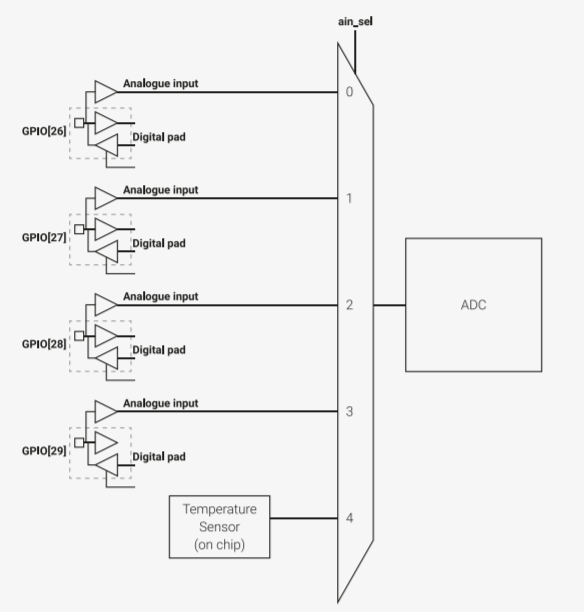uPesy only delivers currently in France.# Measure an analog voltage with the ADC of the Pi Pico in MicroPython

On the Raspberry Pi Pico, some pins are attached to the built-in ADC of the microcontroller. An ADC gives a digital value proportional to the measured input voltage.

Note

ADC stands for Analog to Digital Converter.

## Features

On the Raspberry Pi Pico pins 26, 27 and 28 can be used as an analog input. The resolution of the measured values ​​is on 16 bits, the values ​​are therefore between 0 and 65535.

Note

Hint

There is a “hidden” analog input in the RP2040 microcontroller which allows to measure directly the temperature of the chip. This is somewhat similar to temperature measurements from computer CPUs. It should not be used to get an accurate room temperature. This input corresponds to the channel  ADC_4  .

Warning

Unlike the Arduino, the Pico analog inputs should not exceed a 3.3V voltage. This could damage or even destroy the ADC inputs. Therefore, it is essential to check not to use a 5V supply voltage to measure the value of a sensor. There are solutions to limit the ADC input voltage to 3.3V.

The analog input voltage is measured using the  ADC  submodule of the  machine  module. Like with PWM, we associate a physical pin with the object  ADC  .

from machine import Pin, ADC

# Pin definitions


Remember to import the ADC submodule.

You can also create an  ADC  object by specifying the ADC channel digit you want to use.

from machine import ADC


Once you have configured an analog input in MicroPython, you can read the analog value using the  read_u16()  function on the Raspberry Pi Pico.

from machine import Pin, ADC


It will be necessary to apply a formula to have value measured in volts:

$V_{volt} = \frac{3.3V}{65535} \times V_{bits}$

## Measure the internal temperature of the Raspberry Pi Pico

The Raspberry Pi Pico has an internal temperature sensor to measure the temperature of the RP2040 chip. Although not very reliable, it does provide insight into the temperature of the CPU (just like on computers). This sensor is directly connected to the  ADC_4  input of the ADC.The internal temperature sensor is positioned on the last pin of the ADC

To measure the temperature, you must:
• Retrieve the raw analog value of the temperature sensor available on channel 4 of the ADC

• Convert this value to voltage

• Use a formula given in the RP240 documentation to convert this voltage to temperature (in ° C)

from machine import ADC
import time

while True:
cpu_temp = 27 - (adc_voltage - 0.706)/0.001721 # Formula given in RP2040 Datasheet
print(cpu_temp)
time.sleep_ms(1_000)


Note

You can see the temperature change by putting your finger on the RP2040 chip.

REPL console output:

32.19402
32.19402
32.66216
31.72584
33.13027
33.59842
34.06656
34.06656
34.53474
34.06656


## Project: Control the intensity of an LED via a potentiometer

We will use PWM and ADC with MicroPython to control the LED brightness.

Schematic:

Note

Consider putting a resistor in series with the LED to prevent burning. (330 Ω, for example)

For reading the knob position of the potentiometer only :

from machine import Pin, ADC
import time

while True:

val = val * (3.3 / 65535)
print(round(val, 2), "V") # Keep only 2 digits

# Wait a bit before taking another reading
time.sleep_ms(100)


To read knob position and change the brightness:

from machine import Pin, ADC, PWM
import time

# Create a PWM object linked to pin 15
pwm_led = PWM(Pin(15,mode=Pin.OUT))
pwm_led.freq(1_000)